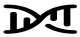# Two-step/Mediation MR

An MR framework that aims to quantify whether a risk factor-outcome association (that is either established or has supportive evidence from MR analyses) is mediated by another risk factor. In many published examples, initial MR will assess evidence for a causal effect of the risk factor on an outcome and then go on to undertake two-step MR. First, genetic IVs associated with the risk factor are used to determine the causal effect of the risk factor on the potential mediator (step one). Secondly, genetic IVs associated with the potential mediator and independent of those used for step one are used to determine the effect of the potential mediator on the outcome of interest (step two). Where evidence is found for a causal effect of a risk factor on an outcome, a risk factor on a mediator and a mediator on an outcome, methods such as multivariable MR or product-of-coefficients can be used to explore the extent of this mediation.

The IV assumptions must be met for the two steps of the MR analyses of the (i) risk factor on mediator and (ii) mediator on outcome. This methodology can be extended to networks of relationships (i.e., network MR).Figure 2.7 - Two-step Mendelian randomization for exploring mediation. (A) In the first step of two-step MR, a genetic variant (Z1) is used as an instrument for the exposure of interest (X) to estimate the causal impact of the exposure on a hypothesized mediator (M) of the association between the exposure (X) and outcome (Y). (B) In the second step, an independent (of Z1) genetic variant (Z2) is used as an instrument for the mediator (M) to establish the causal impact of the mediator (M) on the outcome (Y). If there is evidence for a causal effect of X on M and M on Y (as well as X on Y), the estimates from these two steps can be combined to provide evidence for or against the mediating role of a variable on the exposure-outcome effect using e.g., multivariable MR.